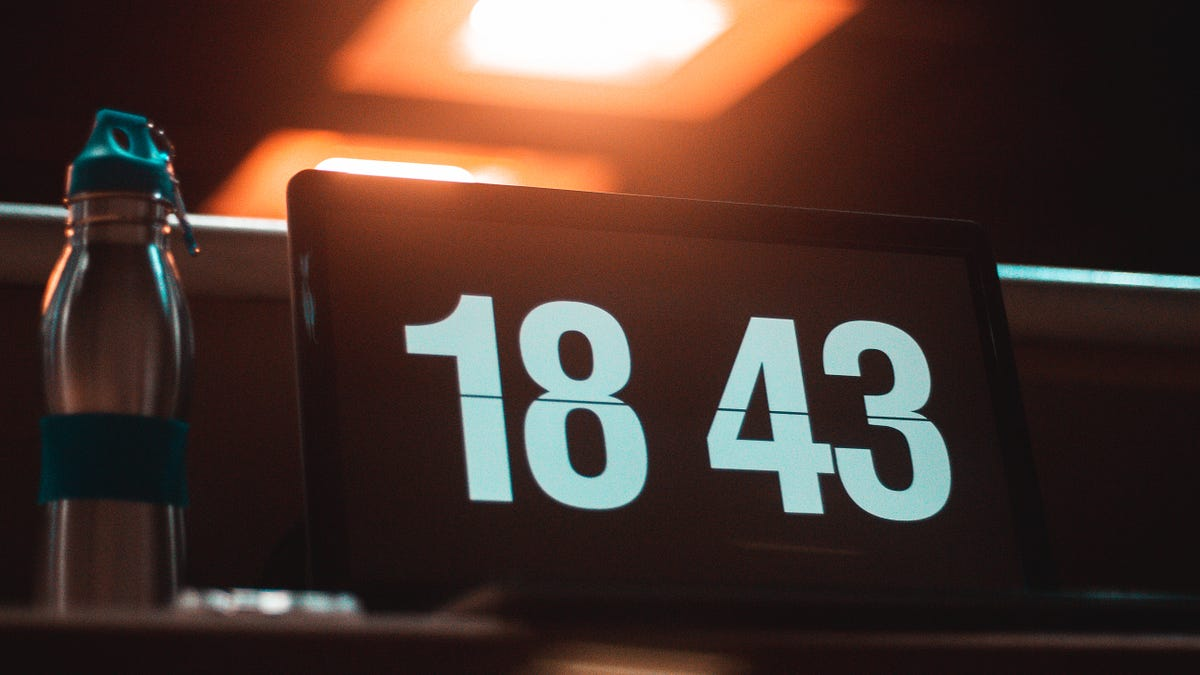# How to make a simple cool looking digital clock with HTML/CSS/JSSo you figured out some HTML and CSS. What’s next? Well, how about making a digital clock!

So you figured out some HTML and CSS. What’s next? Well, how about making a digital clock!

### 1. Index.html

But first, we need an `index.html`file. Make a new `index.html` file and add the code below.

``````<!DOCTYPE html>
<html>
<script src="script.js" async></script>
<div class="line">
<div class="time">
ERROR
ERROR
</div>
</div>
</body>
</html>``````

### 2. Script.js

We’ve added the skeleton but the clock is useless without actually telling the time. Make a new file called `script.js` and add the code below.

``````function startTime() {
var today = new Date();
var h = today.getHours();
var m = today.getMinutes();
var s = today.getSeconds();
m = checkTime(m);
s = checkTime(s);
document.getElementById("time").innerHTML = h + ":" + m + ":" + s; //Add Time to id="time"
var t = setTimeout(startTime, 500);
n = new Date();
y = n.getFullYear();
m = n.getMonth() + 1;
d = n.getDate();
document.getElementById("date").innerHTML = m + "•" + d + "•" + y; //Add Date to id="date"
}

function checkTime(i) {
if (i < 10) {
i = "0" + i;
}
return i;
}
setInterval(() => {
document.title = new Date().toLocaleTimeString(); //Update title tag
}, 1);``````

## Build Digital Clock with JavaScript - Beginner HTML, CSS & JS Tutorial

We'll be building a simple Digital Clock using only HTML, CSS and JavaScript. This demonstrates how we can use web languages/tech to build something tangible.